Ex 7.6

Chapter 7 Class 6 Fractions
Serial order wise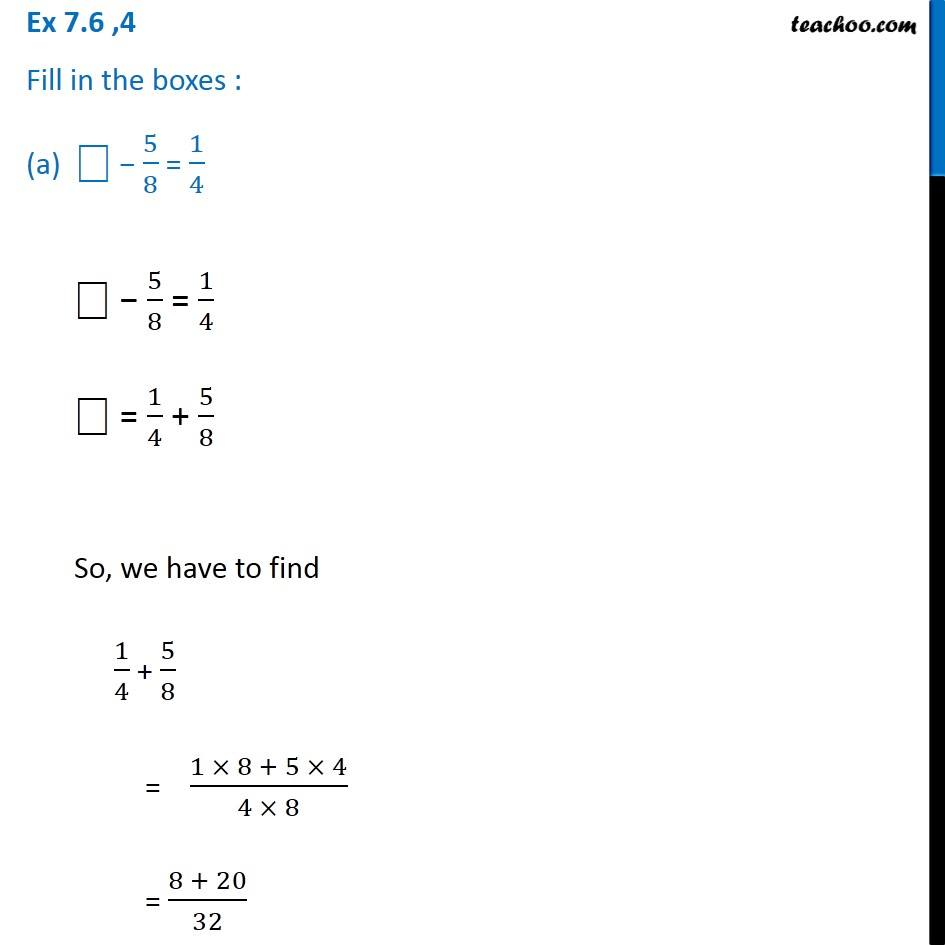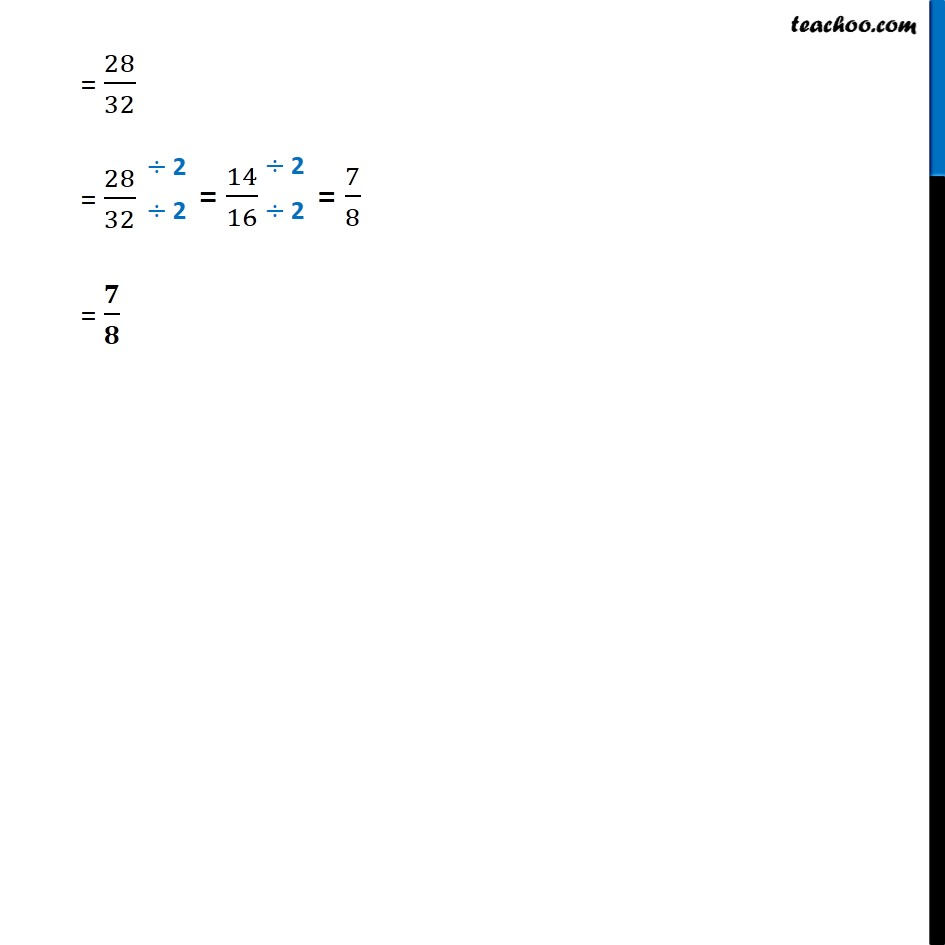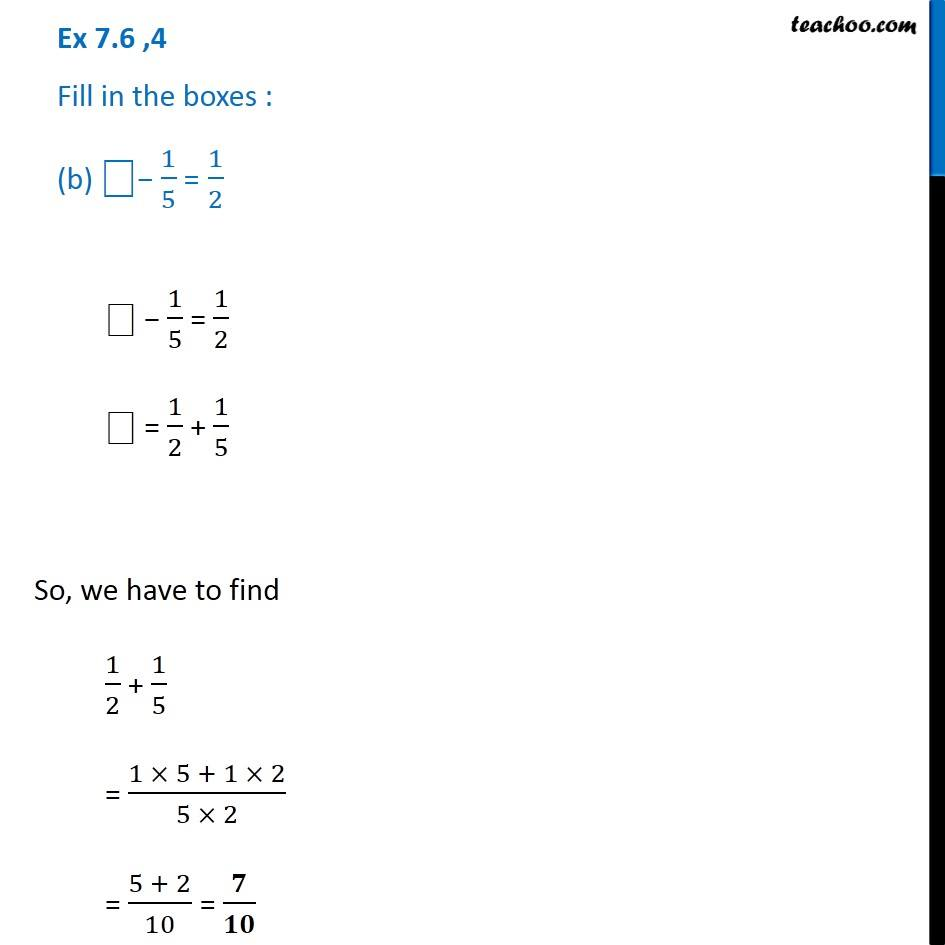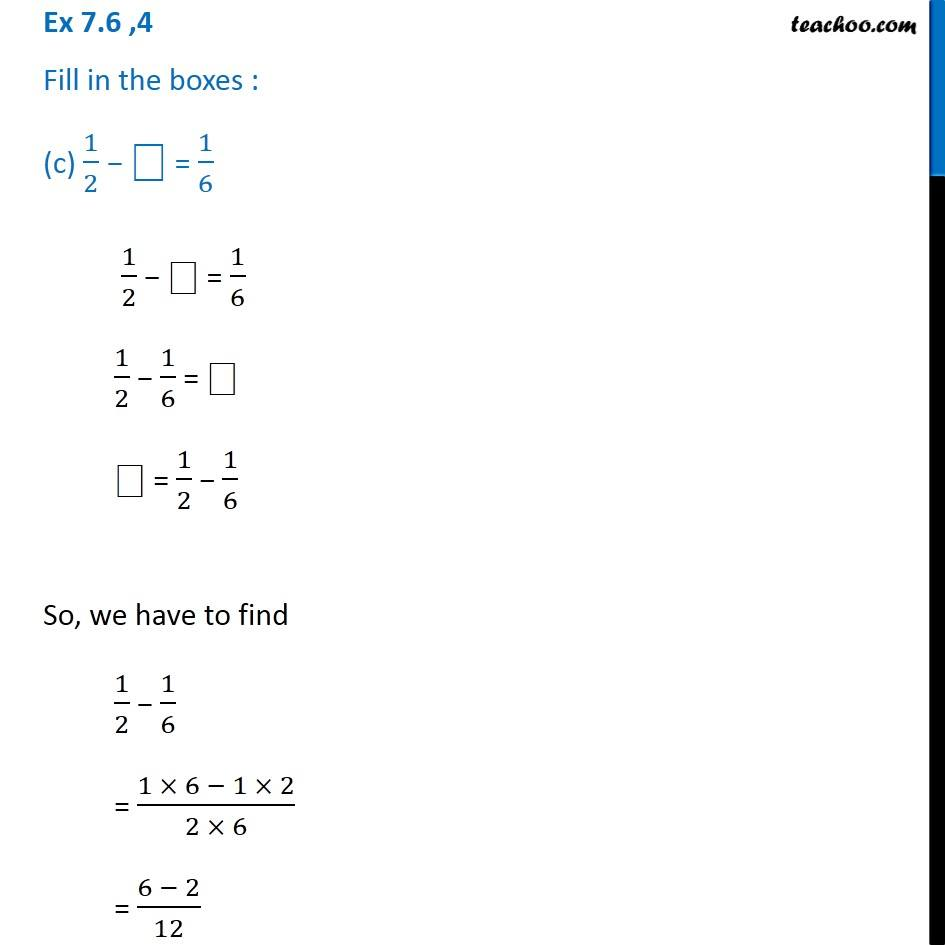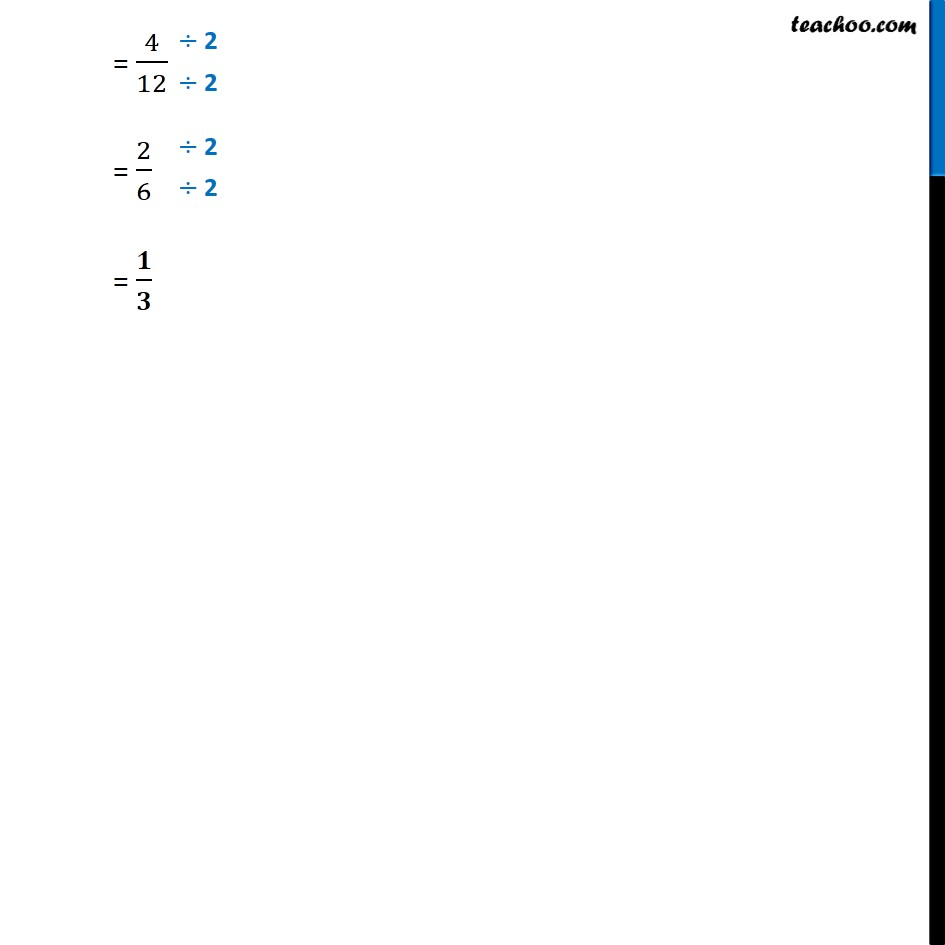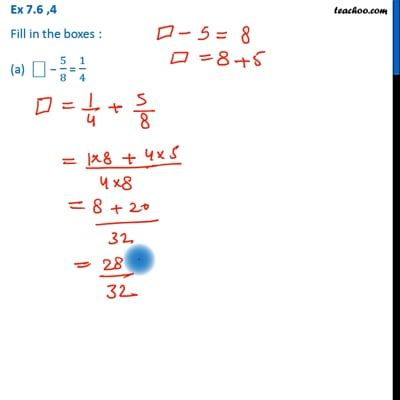This video is only available for Teachoo black users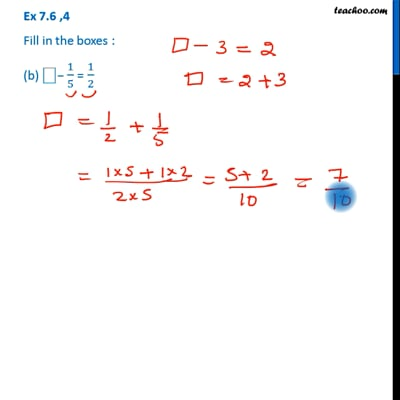This video is only available for Teachoo black users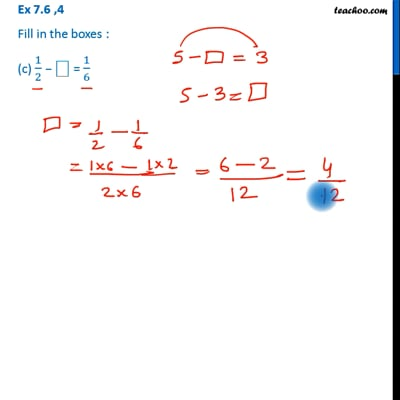This video is only available for Teachoo black users

Get live Maths 1-on-1 Classs - Class 6 to 12

### Transcript

Ex 7.6 ,4 Fill in the boxes : (a) ⎕ − 5/8 = 1/4 ⎕ − 5/8 = 1/4 ⎕ = 1/4 + 5/8 So, we have to find 1/4 + 5/8 = (1 × 8 + 5 × 4)/(4 × 8) = (8 + 20)/32 = 28/32 = 28/32 = 𝟕/𝟖 Ex 7.6 ,4 Fill in the boxes : (c) 1/2 − ⎕ = 1/6 1/2 − ⎕ = 1/6 1/2 − 1/6 = ⎕ ⎕ = 1/2 − 1/6 So, we have to find 1/2 − 1/6 = (1 × 6 − 1 × 2)/(2 × 6) = (6 − 2)/12 = 4/12 = 2/6 = 𝟏/𝟑